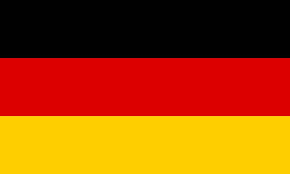Enter the coordinates of for points. Mathepower calculates what (if any) quadrangle they form
A(||)

B(||)

C(||)

D(||)

Enter for points in the plane. Mathepower calculates what quadrangle they form.
A(|)

B(|)

C(|)

D(|)

 Calculating a quadrangle. Enter five arbitrary fields. Other fields will be calculated. a: b: c: d: alpha beta gamma delta Diagonal e: Diagonal f:

## How to find out what quadrangle you got via vector analysis?

Calculate the vectors corresponding to the sides. If opposing vectors are equal, the quadrangle is a rhomboid. Then check for equal length and orthogonality.